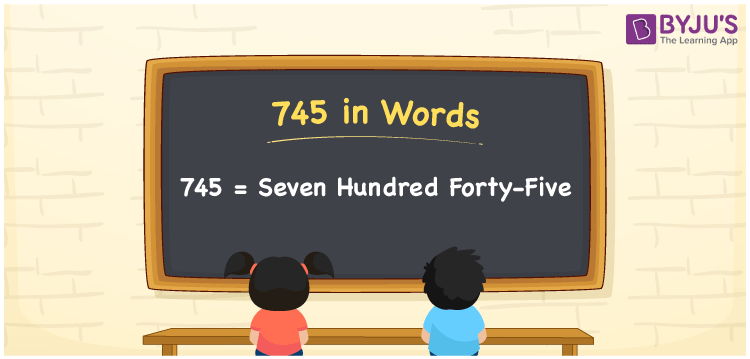# 745 in Words

We can write the number 745 in words as Seven hundred forty-five. If you purchased a playing toy at Rs. 745, then you can say, “I purchased a playing toy for Seven hundred forty-five rupees”. Here, Seven hundred forty-five is the word form or number name for 745. Also, note that 745 is a cardinal number as it denotes a specific quantity.

 745 in words Seven hundred forty-five Seven hundred forty-five in Numbers 745

## 745 in English Words

We generally use the English alphabet to write numbers in words. Thus, we spell 745 in English words as “Seven hundred forty-five”.## How to Write 745 in Words?

The below table displays the place values for all digits of the number 745. Using this table, we can obtain the word form of the number 745.

 Hundreds Tens Ones 7 4 5

Here, ones = 5, tens = 4, hundreds = 7

The above digits can be expanded as follows.

7 × Hundred + 4 × Ten + 5 × One

= 7 × 100 + 4 × 10 + 5 × 1

= 700 + 40 + 5

= Seven hundred + Forty + Five

= Seven hundred forty-five

Therefore, 745 in words is written as Seven hundred forty-five.

745 is a natural number that precedes 746 and succeeds 744.

745 in words – Seven hundred forty-five

Is 745 an even number? – No

Is 745 an odd number? – Yes

Is 745 a prime number? – No

Is 745 a composite number? – Yes

Is 745 a perfect square number? – No

Is 745 a perfect cube number? – No

## Frequently Asked Questions on 745 in Words

Q1

### How do you say 745 in words?

You can spell the number 745 in English words as Seven hundred forty-five.
Q2

### What is 745 in word form?

Seven hundred forty-five is equal to the number 745 in word form.
Q3

### How to write Rs. 745 in words on a cheque?

On a cheque, we generally write an amount of Rs. 745 in words as “Seven hundred forty-five rupees only”.